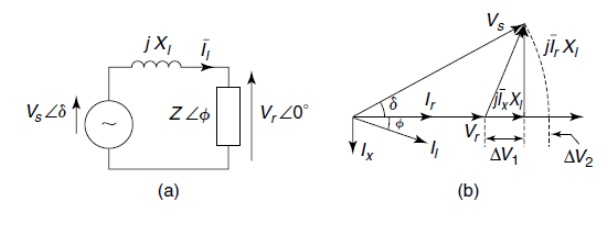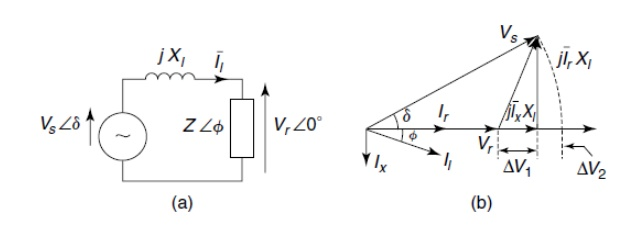Home | | Flexible AC Transmission Systems | Uncompensated Transmission Lines

# Uncompensated Transmission Lines

Two compensation methods are: 1. Load compensation 2. System compensation

UNCOMPENSATED TRANSMISSION LINES

1. Introduction

For simplicity let us consider only the inductive reactanceFrom the above figure it is clear that between he sending and the receiving end voltages and magnitude variation as well as a phase difference is created and the most significant part of the voltage drop in the line reactance is due to the reactive component of the load current and to keep the voltages in the network nearly at the rated value.

Two compensation methods are:

2.     System compensation

Ø   It is possible to compensate for the reactive current of the load by adding a parallel capacitive load so that Ic = Ix and the effective power factor to become unity.

Ø   In the figure the absence of Ix eliminates the voltage drop ∆V1 bringing Vr closer in magnitude to Vs, this condition is called load compensation and actually by charging extra for supplying the reactive power a power utility company makes it advantageous for customers to use load compensation on their premises.

Ø   Loads compensated to the unity power factor reduce the line drop but do not eliminate it. They still experience a drop of ∆V2 from j IrX1.3. System compensation

Ø   To regulate the receiving-end voltage at the rated value a power utility may install a reactive-power compensator as shown in the figure and this compensator draws a reactive current to overcome both components of the voltage drop ∆V1 and ∆V2 as a consequence of the load current I1 through the line reactance X1.

Ø   To compensate for ∆V2 an additional capacitive current ∆Ic over and above Ic that compensates for Ix is drawn by the compensator.

Ø   When ∆Ic X1 = ∆V2 the receiving end voltage Vr equals the sending end voltage Vs and such compensators are employed by power utilities to ensure the quality of supply to their customers.

4. Lossless Distributed Parameter Lines

Ø   Most power transmission lines are characterized by distributed parameters: Series Resistance, Series Inductance, Shunt Conductance and Shunt Capacitance all per-unit length and these parameters all depend on the conductor size, spacing, and clearance above the ground, frequency and temperature of operation.

Ø   In addition these parameters depend on the bundling arrangement of the line conductors and the nearness to other parallel lines.

5. Symmetrical Lines

Ø   When the voltage magnitudes at the two ends of a line are equal that is

Vs = Vr =V and the line is said to be symmetrical because power networks operate as voltage sources attempts are made to hold almost all node voltages at nearly rated values. Therefore a symmetrical line presents a realistic situation.

Ø   Active and Reactive powers of a transmission line are frequently normalized by choosing the Surge-Impedance Load (SIL) as the base.

6. Midpoint Conditions of a Symmetrical Line

Ø   The magnitude of the midpoint voltage depends on the power transfer and this voltage influences the line insulation.

Ø   For a symmetrical line where the end voltages are held at nominal values the midpoint voltage shows the highest magnitude variation.

Study Material, Lecturing Notes, Assignment, Reference, Wiki description explanation, brief detail
Flexible Alternating Current Transmission System : Introduction : Uncompensated Transmission Lines |